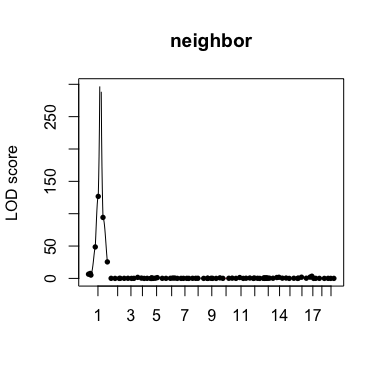# rNeighborQTL

## Overview

The “rNeighborQTL” package includes core functions to perform QTL mapping of neighbor effects. Taking conditional genotype probabilities from the “R/qtl” package (Broman et al. 2003), the “scan_neighbor()” calculates neighbor genotypic identity and performs interval mapping of neighbor QTL effects. The neighbor QTL requires spatial information, namely individual positions along the x- and y-axes, in addition to the genotype and phenotype data. See Sato, Takeda & Nagano (2021) for the theoretical background.

## Input files

First, let us prepare input data using the “R/qtl” package (Broman et al. 2003). Here is an example to import .csv files into a ‘cross’ object and calculate conditional self-genotype probabilities. In this example, we import insect herbivory data on Col x Kas recombinant inbred lines (RILs) of Arabidopsis thaliana (Wilson et al. 2001; Sato, Takeda & Nagano 2021).

colkas <- qtl::read.cross(format="csvs",dir="../inst",
genfile="ColKas_geno.csv",
phefile = "ColKas_pheno.csv",
na.strings = c("_"), estimate.map=TRUE, crosstype = "riself"
)
#>   126  individuals
#>   26  markers
#>   10  phenotypes
#>  --Estimating genetic map
#>  --Cross type: riself

colkas_genoprob <- qtl::calc.genoprob(colkas, step=2)

## Proportion of variation explained by neighbor effects

Prior to the genome scan, we estimate the ‘scale’ argument. Using linear mixed models implemented in the “gaston” package (Perdry & Dandine-Roulland 2020), the “calc_pve()” computes proportion of phenotypic variation (PVE) by neighbor effects for a series of spatial scales. Based on the PVE, we calculate $$\Delta$$PVE metric and seek the scale $$s$$ that gives an argument for the maximum of $$\Delta$$PVE.

library(rNeighborQTL)
x <- colkas$pheno[,2] y <- colkas$pheno[,3]
smap_colkas <- data.frame(x,y)

s_seq <- quantile(dist(smap_colkas),c(0.1*(1:10)))
colkas_pve <- calc_pve(genoprobs=colkas_genoprob,
pheno=log(colkas$pheno[,5]+1), smap=smap_colkas, s_seq=s_seq, addcovar=as.matrix(colkas$pheno[,7:9])
)
#> scale = 2.236
#> scale = 3.162
#> scale = 4.243
#> EM step failed to improve likelihood (this should not happen)
#> scale = 5.099
#> scale = 6
#> scale = 7
#> scale = 7.81
#> scale = 8.602
#> scale = 10.05
#> scale = 15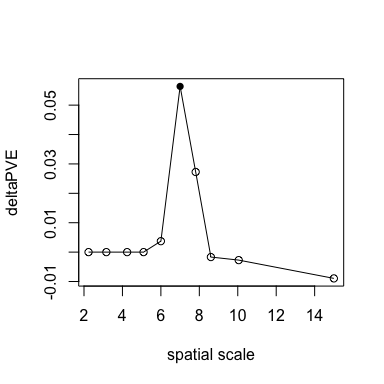## Estimation of QTL effects

Similar to Haley-Knott regression (Haley & Knott 1992), the additive and dominance deviation $$a$$ and $$d$$ are estimated using a linear or quadratic regression on neighbor genotypic identity. The “eff_neighbor()” estimates the coefficients for self and neighbor effects, and plots the results as follows.

colkas_eff <- eff_neighbor(genoprobs=colkas_genoprob,
pheno=log(colkas$pheno[,5]+1), smap=smap_colkas, scale=7, addcovar=as.matrix(colkas$pheno[,7:9])
)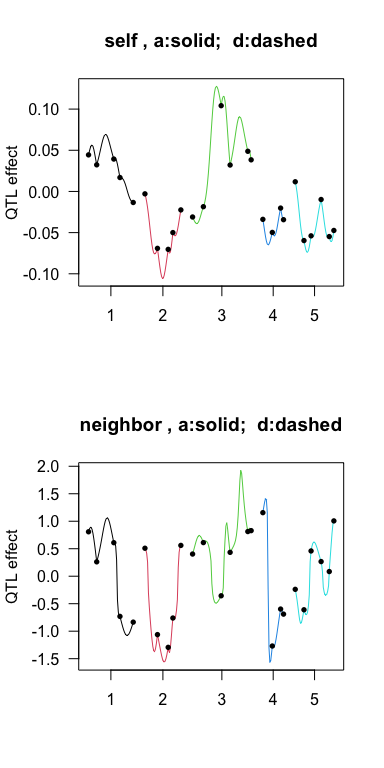## LOD score

Lastly, we perform a genome scan to obtain LOD scores for neighbor QTL effects. The “scan_neighbor()” calculates likelihoods using the estimated QTL effects through the “eff_neighbor()”. The results are drawn by “plot_nei()”.

colkas_scan <- scan_neighbor(genoprobs=colkas_genoprob,
pheno=log(colkas$pheno[,5]+1), smap=smap_colkas, scale=7, addcovar=as.matrix(colkas$pheno[,7:9])
)
plot_nei(colkas_scan)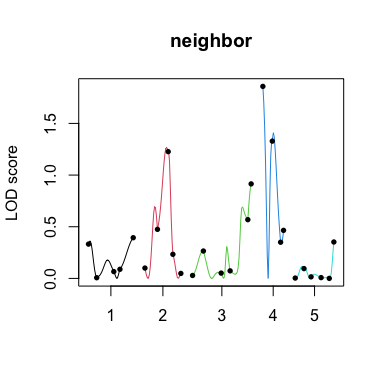In addition to the genome scan, we can perform permutation tests to estimate a genome-wide significance level. Such permutation tests better account data structure, but require much computational time. This is an example code for 3-times permutations.

colkas_perm <- perm_neighbor(genoprobs=colkas_genoprob,
pheno=log(colkas$pheno[,5]+1), smap=smap_colkas, scale=7, addcovar=as.matrix(colkas$pheno[,6:8]),
times=3, p_val=c(0.5,0.1)
)

## Extensions

### 1. Self-genotype effects

The “scan_neighbor()” also provides LOD scores for self QTL effects. This gives the same results as the Haley-Knott regression of standard QTL mapping.

plot_nei(colkas_scan, type="self")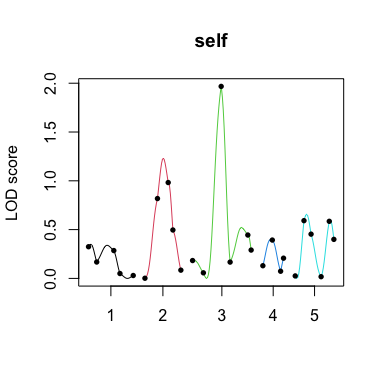colkas_scanone <- qtl::scanone(colkas_genoprob,
pheno.col=log(colkas$pheno$holes+1),
addcovar=as.matrix(colkas$pheno[,7:9]), method="hk") plot(colkas_scanone)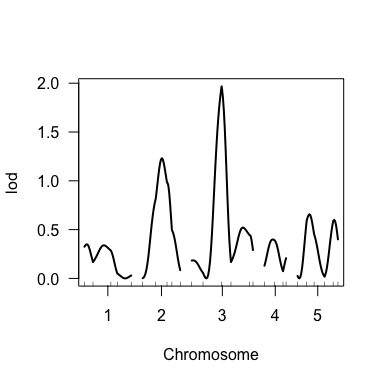### 2. Composite interval mapping The “addQTL” argument allows us to include non-focal QTLs as covariates. This option enables composite interval mapping (Jensen et al. 1993) that considers additional QTL effects. Here is an example code using the Col x Kas herbivory data, with the nga8 marker considered a covariate. colkas_cim <- scan_neighbor(genoprobs=colkas_genoprob, pheno=log(colkas$pheno[,5]+1),
smap=smap_colkas, scale=7,
addcovar=as.matrix(colkas$pheno[,7:9]), addQTL="c4_nga8" ) plot_nei(colkas_cim) ### 3. Epistasis in neighbor QTL effects For the analysis of epistasis, the “int_neighbor()” calculate LOD score of two-way interactions between a focal marker and the others. Here is an example code for the ‘nga8’ marker in the Col x Kas herbivory data. colkas_int <- int_neighbor(genoprobs=colkas_genoprob, pheno=log(colkas$pheno[,5]+1),
smap=smap_colkas, scale=7,
addcovar=as.matrix(colkas$pheno[,7:9]), addQTL="c4_nga8", intQTL="c4_nga8" ) plot_nei(colkas_int, type="int") ### 4. Binary traits The “response” argument allows us to analyze “binary” phenotypes as well as “quantitative” traits. This argument calls logistic (mixed) models internally (Faraway 2016; Chen et al. 2016). The “calc_pve()” yields the ratio of phenotypic variation explained (RVE) by neighbor effects as RVEnei =$$\sigma^2_2/\sigma^2_1$$ when “binary” traits are analyzed, because the logistic mixed model does not compute $$\sigma^2_e$$ (Perdry & Dandine-Roulland 2020). Here is an example code for the analysis of the presence or absence of bolting in Col x Kas RILs. s_seq <- quantile(dist(smap_colkas),c(0.1*(1:10))) colkas_pveBin <- calc_pve(genoprobs=colkas_genoprob, pheno=colkas$pheno[,7],
smap=smap_colkas, s_seq=s_seq,
response="binary", addcovar=as.matrix(colkas$pheno[,8:9]), fig=TRUE) #> scale = 2.236 #> scale = 3.162 #> scale = 4.243 #> scale = 5.099 #> scale = 6 #> scale = 7 #> scale = 7.81 #> scale = 8.602 #> scale = 10.05 #> scale = 15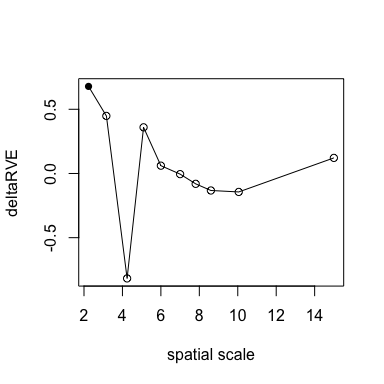colkas_scanBin <- scan_neighbor(genoprobs=colkas_genoprob, pheno=colkas$pheno[,7],
smap=smap_colkas, scale=2.24,
addcovar=as.matrix(colkas$pheno[,8:9]), response="binary") plot_nei(colkas_scanBin)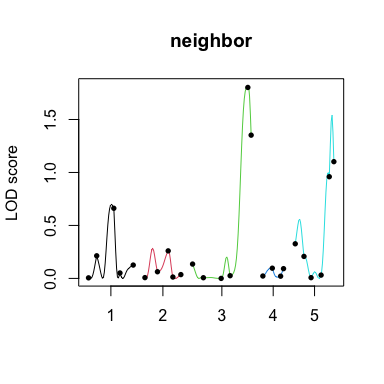### 5. Crossing design The neighbor QTL package is able to handle AB heterozygotes. It also works even when there are only AA or AB genotypes. However, sex chromosomes are not supported currently, and should be excluded before the genome scan. This is a simulation using F2 or backcross lines implemented in the “R/qtl” package. #F2 lines set.seed(1234) data("fake.f2",package="qtl") fake_f2 <- subset(fake.f2, chr=1:19) smap_f2 <- cbind(runif(qtl::nind(fake_f2),1,100),runif(qtl::nind(fake_f2),1,100)) genoprobs_f2 <- qtl::calc.genoprob(fake_f2,step=2) s_seq <- quantile(dist(smap_f2),c(0.1*(1:10))) nei_eff <- sim_nei_qtl(genoprobs_f2, a2=0.5, d2=0.5, smap=smap_f2, scale=s_seq, n_QTL=1 ) pve_f2 <- calc_pve(genoprobs=genoprobs_f2, pheno=nei_eff$nei_y,
smap=smap_f2, s_seq=s_seq[1:5],
addcovar=as.matrix(cbind(fake_f2$pheno$sex,fake_f2$pheno$pgm)),
fig=FALSE)
#> scale = 19.368
#> scale = 28.245
#> scale = 35.695
#> scale = 42.909
#> scale = 49.961

deltaPVE <- pve_f2[-1,3] - c(0,pve_f2[1:4,3])
argmax_s <- s_seq[1:5][deltaPVE==max(deltaPVE)]

scan_f2 <- scan_neighbor(genoprobs=genoprobs_f2,
pheno=nei_eff$nei_y, smap=smap_f2, scale=argmax_s, addcovar=as.matrix(cbind(fake_f2$pheno$sex,fake_f2$pheno$pgm)) ) plot_nei(scan_f2)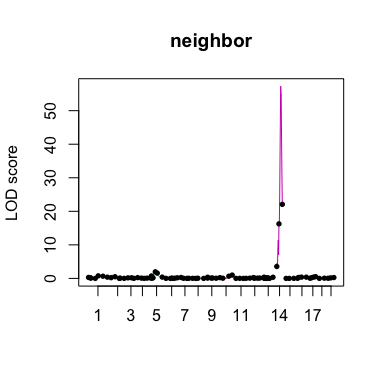#backcross lines set.seed(1234) data("fake.bc",package="qtl") fake_bc <- subset(fake.bc, chr=1:19) smap_bc <- cbind(runif(qtl::nind(fake_bc),1,100),runif(qtl::nind(fake_bc),1,100)) genoprobs_bc <- qtl::calc.genoprob(fake_bc,step=2) s_seq <- quantile(dist(smap_bc),c(0.1*(1:10))) nei_eff <- sim_nei_qtl(genoprobs_bc, a2=0.3, d2=-0.3, smap=smap_bc, scale=s_seq, n_QTL=1) pve_bc <- calc_pve(genoprobs=genoprobs_bc, pheno=nei_eff$nei_y,
smap=smap_bc, s_seq=s_seq[1:5],
addcovar=as.matrix(cbind(fake_bc$pheno$sex,fake_bc$pheno$age)),
fig=FALSE)
#> scale = 19.256
#> scale = 28.487
#> scale = 36.266
#> scale = 43.479
#> scale = 50.618

deltaPVE <- pve_bc[-1,3] - c(0,pve_bc[1:4,3])
argmax_s <- s_seq[1:5][deltaPVE==max(deltaPVE)]

scan_bc <- scan_neighbor(genoprobs=genoprobs_bc,
pheno=nei_eff$nei_y, smap=smap_bc, scale=argmax_s, addcovar=as.matrix(cbind(fake_bc$pheno$sex,fake_bc$pheno\$age))
)

plot_nei(scan_bc)